# Population dynamics

## Lotka-Volterra two-species competition model

ModelId:
competition1
SimileVersion:
3.1+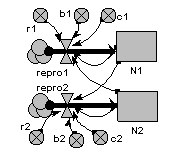This is the classic textbook model of the population dynamics of two competing species.

It is based on a pair of differential equations, each having the same mathematical form:

dX1/dt = r1.X1.(1-b1.X1-c1.X2)
dX2/dt = r2.X2.(1-b2.X2-c2.X1)

Results: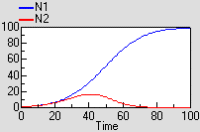## Skeleton cohort model

ModelId:
cohort1
SimileVersion:
5.97

This example shows how to implement a basic cohort model in Simile.

A cohort model is one way of modelling a population. The individuals are grouped into cohorts, typically on the basis that they were born at the same time. Each cohort has as a minimum a variable representing the number of individuals in that cohort, along with possibly other cohort-specific attributes, such as the average size of the individuals in the cohort.

Equations:

Variable: n_total = sum({n})

Where: {n} = Cohort/n

Variable: population_total_size = sum({cohort_total_size})
Where: {cohort_total_size} = Cohort/cohort_total_size

--------------------------------------------------------------
Submodel Bigger

Submodel "Bigger" is an association submodel between "Cohort" and itself with roles "other" and "me".

Condition: condition = other_size>me_size
Where:
me_size = Value(s) of ../Cohort/size from submodel "Cohort" in role "me"
other_size = Value(s) of ../Cohort/size from submodel "Cohort" in role "other"

Variable: total_size_of_bigger_cohort = other_cohort_total_size
Where:
me_cohort_total_size = Value(s) of ../Cohort/cohort_total_size from submodel "Cohort" in role "me"
other_cohort_total_size = Value(s) of ../Cohort/cohort_total_size from submodel "Cohort" in role "other"

-------------------------------------------------------------------------
Submodel Cohort

Submodel "Cohort" is a population submodel.

Compartment: n
Initial value = 100
Rate of change = - loss

Compartment: size
Initial value = 0
Rate of change = + growth

Flow: growth = gr*(1-size/max_size)

Flow: loss = 5

Immigration: create_new_cohort = 1

Loss: remove_cohort = n<=0

Variable: cohort_total_size = n*size

Variable: gr = 1.5

Variable: max_size = 10

Variable: total_size_of_all_bigger_cohorts = sum({cohort_total_size_of_bigger_me})
Where:
{cohort_total_size_of_bigger_me} = ../Bigger/total_size_of_bigger_cohort for submodel "Cohort" in role "me"

Results:

The results shown here illustrate the basic functioning of this model.

The following figure shows a set of panels, each one showing the values for a specified variable at time 10. These were made using Simile's 'snapshot' tool, and enable us to trace the values as they are processed in the model.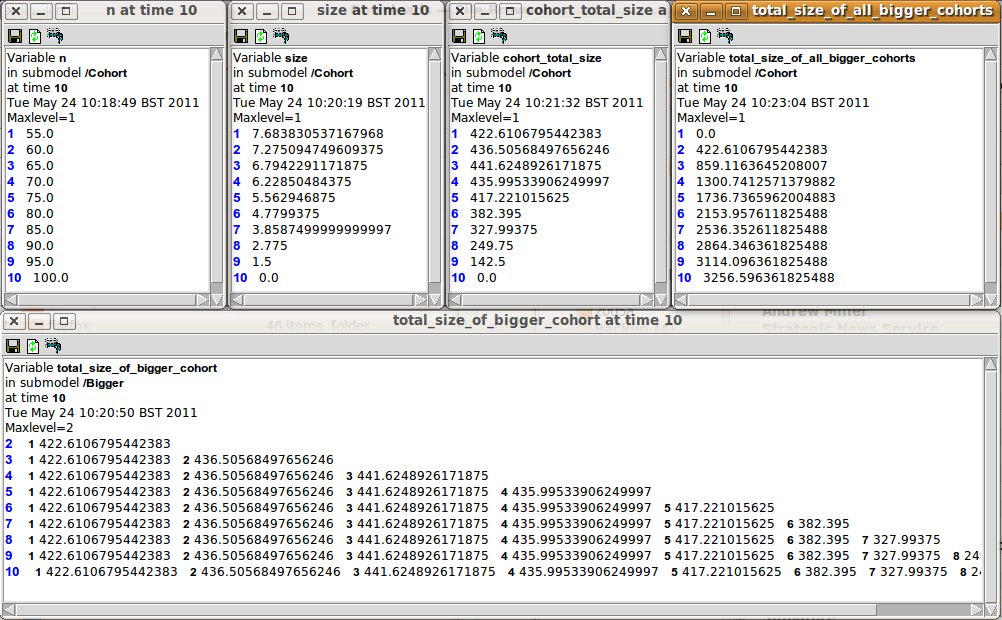The first 3 panels on the top row show, for each of the 10 cohorts created up to this time, the number of tress (n) and the size of the average tree (size) in the cohort, along with the total size (n x size). You can see that the number of tress declines in a cohort declines by 5 each time step, and that the average tree in a cohort grows at a reducing rate as the cohort gets older.

The bottom window shows the total_size values for the individual cohorts that are bigger than the specified cohort. Thus, cohort 2 only has one value (since there is only one bigger than it is), while cohort 3 has 2 values, and so on.

The top-right window shows 'total_size_of_all_bigger_cohorts' - the sum (along a row) for al the total_size values of bigger cohorts for a particular cohort. This is the key value which enables the impact of bigger cohorts on the growth of a cohort to be modelled.

The graph below shows how the 'total_size_of_all_bigger_cohorts' changes over time, for each cohort. The lowest curve is for the second cohort (since the first cohort has nothing bigger than it. Each successive cohort starts off at a higher value (since the total size of cohorts above it is increasing), until we get to the point where each newly-created cohort starts off with the same total_size above it, which decreases as that cohort in turn increases in size.## Population dynamics: single species: chaotic

ModelId:
chaos
SimileVersion:
5.x

This version differs from the standard population model in two respects:

Equations:

Compartment level

Initial value = rand_const(50,50.000001)
Rate of change = + change

Flow change
change = gain*level*(1-level/100)

﻿

Results:

This graph shows the results of four consecutive runs with gain set to 1.4, 1.9, 2.3 and 2.7 respectively. On the first two runs the level approaches equilibrium; on the third it oscillates around the equilibrium, and on the final run it shows chaotic behaviour. Other behaviours such as period doubling will be shown for other values of gain.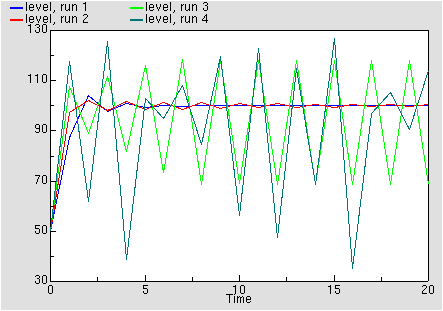This graph illustrates the unpredictability of chaotic behaviour. The model includes a very small random variation in the initial value of level, but the values for three consecutive runs, all with gain set to 2.7, diverge after about 60 time units. Until this time, the plot for the last run (green) exactly overwrites the other two.

## Budworm population dynamics

ModelId:
budworm1
SimileVersion:
5.x

This is a simple, two-compartment model of the interaction between a spruce budworm population and the forest resource (foliage biomass) that it depends upon. As the budworm population expands, it tends to eat out the foliage, leading to a population crash and the eventual recovery of the forest.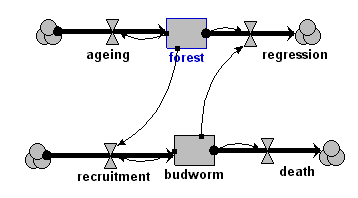Equations:

Compartments:

forest: initial value = 1
budworm: initial value = 10

Flows:
ageing = (if forest<4 then 0.02 else 0)
regression = (if budworm>300 then forest-1 else 0)
recruitment = forest*graph(0,400,400,5,0,400,1,21,points(292,315,346,346,328,311,302,301,301,308,318,327,332,339,345,346,348,352,352,352,353),budworm)*budworm where[budworm=budworm,forest=forest]

Model tags:

## Individual-based model of population dynamics with movement of individual animals

ModelId:
animal1
SimileVersion:
5.9

This models the dynamics of a population of animals, represented as a set of individuals that come into existence, live for a while, then die. When the animals are alive, they move around according to a simple random walk.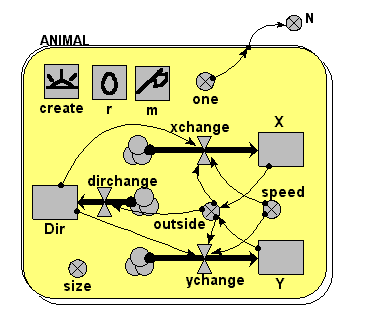Equations:

Equations in Desktop

N = sum({one})

Equations in ANIMAL
X: initial value = rand(30,70)
Y: initial value = rand(30,70)
Dir: initial value = rand(0,6.28)

create = 100
r = rand(0,2*0.7)
m = 0.6

xchange = (if outside == 1 then speed*cos(dir+3.14) else speed*cos(dir))
dirchange = (if outside == 1 then 3.14/dt(1) else rand(-0.4,0.4)/dt(1))
ychange = (if outside == 1 then speed*sin(dir+3.14) else speed*sin(dir))

speed = 5
size = 3
outside = (if (x<0;x>100;y<0;y>100)then 1 else 0)
one = 1

Results: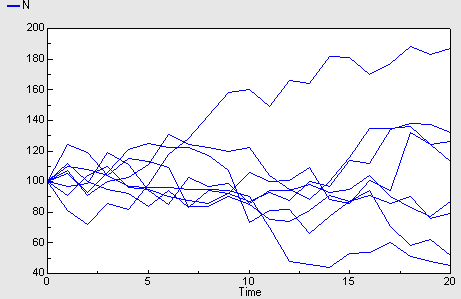Model tags:

## Age-class model of population dynamics, using a multiple-instance submodel and an association submodel

ModelId:
ageclass6
SimileVersion:
5.9

This models a population of animals in terms of four age-classes. For each age-class, we use a compartment (state variable) to represent the number of individuals in that class. This version uses a four-instance submodel to represent the four age-classes. The ageing flow (from one class to the next) thus has to be handled by transferring the value for the outflow from each class to be assigned to the inflow for the next class.

Equations:

Equations in Desktop

births = sum([these_births])
pop size = sum([pop_size])

Equations in Age class

pop size: initial value = element([2,0,0,0],index(1))

ageing in = sum({ageing_next})
Where: {ageing_next}=../Next class/ageing (to Age class in next)

ageing out = element([1,0.2,0.1,0],index(1))*pop_size

births = if index(1)==1 then births else 0

deaths = m*pop_size

class = index(1)

m = element([0.05,0.01,0.01,0.05],index(1))

r = element([0,0.05,0.2,0.1],index(1))*(1-total_pop_size/100)

these births = r*pop_size

Equations in Next class

condition = class_next==class_this+1
Where: class_this=../Age class/class (from Age class in this) class_next=../Age class/class (from Age class in next)

ageing = ageing_out_this
Where: ageing_out_this=../Age class/ageing out (from Age class in this)

here: ageing_out_this=../Age class/ageing out (from Age class in this)/

Results: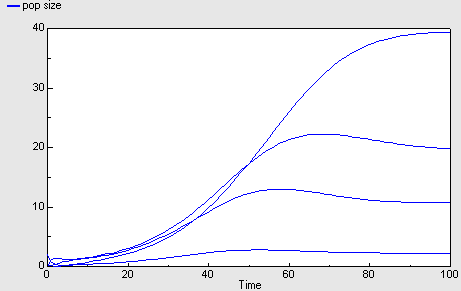## Age-class model of population dynamics, using multiple-instance submodel

ModelId:
ageclass5
SimileVersion:
5.9

This models a population of animals in terms of four age-classes. For each age-class, we use a compartment (state variable) to represent the number of individuals in that class.

Equations:

Equations in Desktop

births = sum([these_births])
pop size = sum([pop_size])
ageing = [ageing_out]

Equations in Age class
pop size: initial value = element([2,0,0,0],index(1))
births = if index(1) == 1 then births else 0
ageing out = element([1,0.2,0.1,0],index(1))*pop_size
deaths = m*pop_size
ageing in = if index(1)>1 then element([ageing],index(1)-1)else 0
m = element([0.05,0.01,0.01,0.05],index(1))
r = element([0,0.05,0.2,0.1],index(1))*(1-pop_size/100)
these births= r*pop_size

Results:Model tags:

## Age-class model of population dynamics, one compartment per age class

ModelId:
ageclass4
SimileVersion:
5.9

This models a population of animals in terms of four age-classes. For each age-class, we use a compartment (state variable) to represent the number of individuals in that class.

Equations:

Compartments

pop_size_1: initial value = 2
pop size_2: initial value = 0
pop size_3: initial value = 0
pop size_4: initial value = 0

Flows
ageing_1 = pop_size_1
ageing_2 = 0.2*pop_size_2
ageing_3 = 0.1*pop_size_3
births = births_2+births_3+births_4
deaths_1 = m1*pop_size_1
deaths_2 = m2*pop_size_2
deaths_3 = m3*pop_size_3
deaths_4 = m4*pop_size_4

Parameters
m1 = 0.05
m2 = 0.01
m3 = 0.01
m4 = 0.05

Intermediate variables
births_2 = r2*pop_size_2
births_3 = r3*pop_size_3
births_4 = r4*pop_size_4
pop size = pop_size_1+pop_size_2+pop_size_3+pop_size_4
r2 = 0.05*(1-pop_size/100)
r3 = 0.2*(1-pop_size/100)

Results: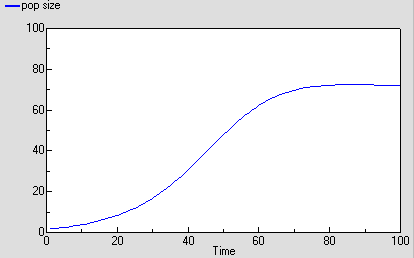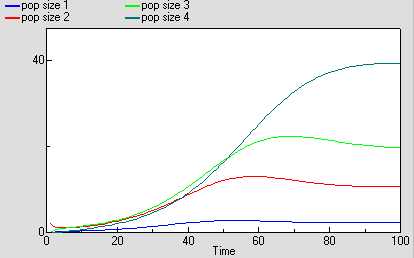Model tags: